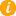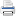Math K-Plus

# Practice 4th Grade Long Multiplication Calculator

TestQuizQuiz Results## Fourth Grade Long Multiplication Calculator

• Option 2. Solve a calculator generated math problem. First press the NEW PROBLEM button. Next enter your answer, in to the calculator. Lastly, press the ADD button and then compare your answer with the calculator's.

Field Name Multiply Values
Multiplicand: You can enter only 5 digits
Multiplier: You can enter only 3 digits
×STEP 18.

29493
×  117
206451
29493
29493
+3450681